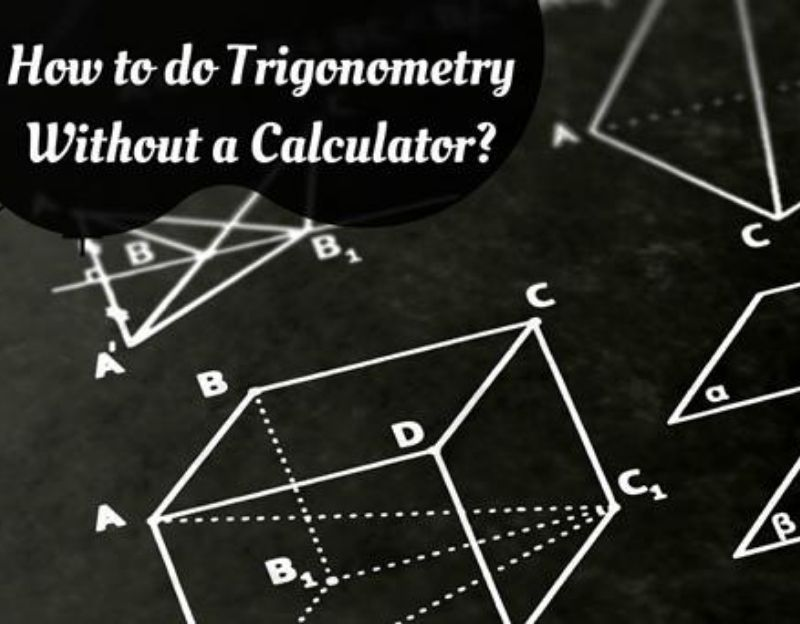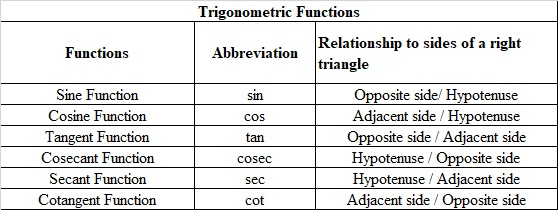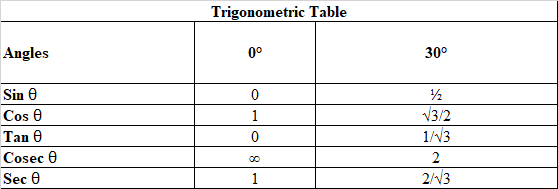# How to Do Trigonometry Without a Calculator

Aug 17, 2020Trigonometry is a branch in mathematics that deals with the study of triangles, and the lengths and angles of their sides. In the real world, trigonometry has its applications into various fields including but not limited to architecture, surveys, astronomy, physics, engineering, and more. It is one of the most applicable subjects in mathematics that has its usage in many occupations.

Solving trigonometric sums on a calculator saves a lot of time and provides an accurate answer. However, trigonometry sums can be solved without the help of a calculator too. And though unbelievable, it's not as hard as it seems to be. All you have to do is use the Trigonometry table and you will be able to crack up most of the answers in a short time and let me tell you that it's even fun. But before moving on to solving trigonometric problems without a calculator, let me guide you through the fundamentals of trigonometry.

### Trigonometry - Fundamentals

There are three primary functions in trigonometry which are sine, cosine, and tangent. It is one of those divisions in mathematics that helps us to find the angles and missing sides of a triangle with the help of trigonometric ratios. The commonly used angles in trigonometry are 0°, 30°, 45°, 60°, and 90°. These trigonometry angles are either measured in radians or degrees.

There are two sub-branches to trigonometry namely plane trigonometry and spherical geometry.

Apart from the three primary functions that are abbreviated as sin, cos, and tan, there are three other trigonometric ratios- cos, cosec, and cot. To know more about how these ratios are defined, let us first understand the sides of the right-angled triangle with an example.Consider a right-angled triangle, where the side opposite to the right angle is called hypotenuse and the other two sides are adjacent and opposite.
Considering these sides the various trigonometric functions are defined as below.### Tricks: SOH CAH TOA

The S in SOH represents Sine, while the O is for Opposite, and the H is for Hypotenuse. Similarly, the other ratios can be remembered. Also if you look at the Trigonometric functions table , it's pretty simple to figure out that cosec, sec, and cot are inverse of sin, cos, and tan respectively.

Now that you are aware of the ratios, there's just one more thing you need to know before solving trigonometry problems. From the above image of a right-angled triangle, it can be seen that

Sin θ = Opposite/Hypotenuse or θ = sin-1 (O/H)
Similarly,
θ = cos-1 (Adjacent/Hypotenuse)
θ = tan-1 (Opposite/Adjacent)

We have accumulated a table for you with the common angles that are used in trigonometry- 0°, 30°, 45°, 60°, and 90°. You can find the values for common angles for the trigonometric ratios in the table given below.Following this table, we can also calculate the trigonometric ratio values for angles beyond 90° such as 180°, 270°, and 360°.

Having knowledge of the above basics of trigonometry is very useful in solving problems. Most of the problems will be solved by just using the tables and simple information we have learned in this article. And the best part is, you don’t need a calculator for it. Try to keep this information at the tip of your tongue so you can solve your trigonometry problems in a few minutes without using any trigonometry calculator.

### Evaluating Trigonometric Functions without a Calculator

For trigonometric functions of Graphical Axes, you can easily solve the problems using the easy-to-remember patterns for 0°, 90°, 180°, and 270°. The values of Sine and Cosine for these angles are quite easy to be saved in your memory. The sine of 0° is 0, the sine of 90° is 1, the sine of 180° is 0 and for 270° the value is -1. The values of cosine are also similar to sine’s cycle, just that it starts at 1. The cosine of 0° is 1, the cosine of 90° is 0, the cosine of 180° is -1 and for 270° the value is 0.

For Right Triangles or right-angled triangles, you have to follow the SOH CAH TOA rule. For example, if you have a right-angled triangle with an acute angle of 30° and a hypotenuse with a length of 5 feet and you have to find the length of the side opposite to the acute angle.

Here, you will have to consider the ratio that relates between the given length and the length that is to be found. So we can use the sine function,

sin x = opposite / hypotenuse

sin 30° = opposite / 5

You can replace the value of sin 30° in this formula from the table given above.

1 / 2 = opposite / 5

Therefore, opposite = 5 / 2 = 2.5

Hence, we can easily find the other angles and sides if we have a few mentioned.

You can also remember the trigonometric values of commonly used angles by using Special Triangles. The 30-60-90 and 45-45-90 are special triangles that are used to remember the trigonometric functions of such commonly used angles. For a 30-60-90 triangle, you have to consider the sides of length 1 (the side opposite to 60°), 2 (hypotenuse), and square root of 3 (the side opposite to 30°). And for a 45-45-90 triangle, you have to consider the sides of length square root 2 (hypotenuse), and the other two sides of length 1. You can then easily find the sine and cosine functions for all these degrees by remembering just these simple special triangles.

The Trigonometric Table is also used in instances when a special angle or a triangle is given. You can use the trigonometric table easily as it has most of the commonly used angles’ values for all the six important trigonometry functions.

Now that we have all the necessary information on trigonometric functions, right triangles, values of trigonometric ratios for common angles, and also the inverse functions, it is really easy to solve trigonometric problems. You won’t need a calculator for it and it is quite simple and interesting too.

Article Posted in: Academics
Tags: Trigonometry### MyPrivateTutor UAE

MyPrivateTutor is an online marketplace for all kinds of tutoring and learning services. We provide a platform to help learners find home tutors, online tutors, expert trainers,tutorial centers, training institutes and online tutoring schools. Learners can also find local classes, workshops, online courses and tutorials on a huge variety of topics. We operate in 12 countries worldwide and our community of learners, teachers and training businesses numbers over 200,000. We provide an opportunity for individual tutors and tutoring businesses to promote and grow their business.